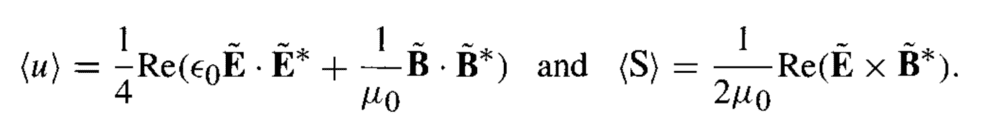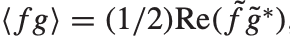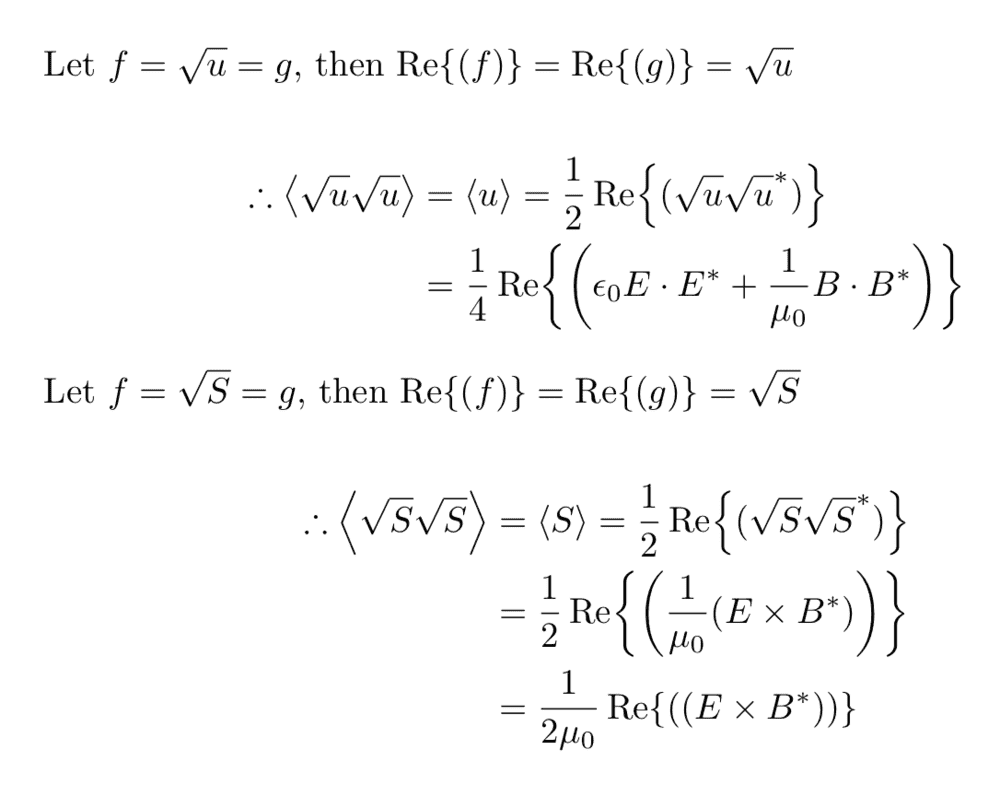# Average Energy density and the Poynting vector of an EM wave

edfink1
Homework Statement:
Average Energy density and the Poynting vector of an EM wave
Relevant Equations:
energy density of the EM fields, Poynting vector
Hi,
In Problem 9.12 of Griffiths Introduction to Electrodynamics, 4th edition (Problem 9.11 3rd edition), in the problem, he says that one can calculate the average energy density and Poynting vector asusing the formulaI don't really understand how to do this. I show my attempt below, but I don't think it is good. Can someone explain if it is really all there is to it?•Delta2